Published: 15 May 2018

# Loss factor estimation of the layered panels

Jan Petřík1
Robert Fiedler2
Petr Lepšík3
1, 3Technical University of Liberec, Liberec, Czech Republic
2MECAS ESI s.r.o., Brojova 2113/16, Plzeň, Czech Republic
Corresponding Author:
Jan Petřík
Views 67

#### Abstract

The loss factor identification is the effective way to get the proper acoustic analysis in the virtual environment. Using the predictive model is a necessary part of the virtual development. The detection of the inappropriate materials or malfunction designs in the early phase of product development and can dramatically save the cost and development time. The loss factor is the nonlinear parameter for the material modeling of the noise suppression during acoustic analysis. The identification of the loss factor is necessary to perform on the real specimen. The Decay Rate Method is the effective methodology to get accurate results by using standard measuring equipment and is ideal to use in-situ environment. The article describes loss factor identification for plywood material and applications for the automotive industry for glass materials. The complete results analysis is confidential, but the overall procedure of the method is described in specimen analysis.

## 1. Introduction

The identification of the structural damping is the most important part of the acoustic and NVH (Noise Vibration Harshness) development. The non-linear behavior of the structural damping causes the low correlation between simulation and real measurements. The analysis also causes the dispersion of the estimated results, and the statistical sample of the measurements needs to be broad as possible.

Over the decades, several methods have been proposed to analyze structural damping behavior of the materials. Oberst Beam Method  was proposed to determine the damping loss factors (DLF), in practice for this method where the specimen is fixed on one side, and another side is excited by an electromagnetic induction. Damping estimation is then related to the identification of the frequency bandwidth using half-power (-3dB) points around the resonances of transmissibility spectrum. The major drawback of this method is that it might be in excitation of non-metallic materials and damping estimation for closely spaced (higher order) modes, where modal overlap affects frequency bandwidth identification. However, this method is restricted to so-called in-situ panels damping estimation, where damping mechanism can be influenced by attached structures, shape, profile, variable sections, and others.

Also, the DLF can also be estimated by the Power Injection Method (PIM) . This technique uses stationary broadband force excitation and set of accelerometers randomly placed on the panel. The loss factor is defined as the ratio of the energy loss per radian to the total energy of the systems. To apply the PIM a stable stationary force exciter needs to be required.

The decay rate method (DRM) is an efficient method, which is based on the domain frequency response of the impact pulse force . Generally, this method relies on the force impulse performed by the hammer and response at mounted accelerometers array randomly installed on the panel(s) or whole structural body. However, this method can identify the in-situ damping as near to the real scenario, nevertheless, there is no influence of the specimen dimension, therefore, the advantage of this method can ensure to provide the accurate results rather than other available methods. During the damping behavior estimation, there is a possibility for the complexity of identified objects which is not a hindrance. While in the practical application, there is a potential problem may arise due to the improper accelerometers sensitivity selection as well as to get the relevant magnitude spectrum on the force sensor which is placed inside ht impact hammer to appropriate signal to noise distance. In most case, the recommended accelerometer should be low weight, therefore it could not be affected by the panel behavior, however, their small weight of the seismic mass causes the signal distortion in the low-band measurement. The charge force sensor can be utilized by the impact hammer; however, it is recommended, due to the increased sensitivity to the shock pulses. The stochastic behavior is shown by the impact hammer impulses; therefore, it is necessary to execute the sufficient number of experiment to make average values for statistical calculations. The response of resonant modes can be utilized to estimate the damping loss factor, but in theory, the all modes of damping can be excited to get correct panel damping, so, it is necessary to estimate randomly on the accelerometer location and its impact on each panel.

The PIM and DRM were used to estimate the damping loss factor. The comparison between the PIM and DRM describes [2, 3] and both methods present good results when there are modes within the analyzed frequency band. It is possible to see that both methods show close results. Although at low frequencies bandwidth, the PIM showed less accurate. The PIM has a strong dependency on the amount of the response points. The DRM method, which uses the initial decay of the impulse response, can be faster than PIM because it requires only a little quantities of frequency response function (FRF). Therefore, the DRM could be limited to apply for lightly damped structures, for DLF < 0.1 (where 1 = 100 %). The limitation is related to frequency bandwidth filter used when filtering FRF to estimate time decay for particular Third Octave Frequency point.

In this paper, the DRM method was chosen for estimating of the DLF of the plywood panels and presents the loss factor identification for one plywood panel with the statistical dispersion of results. The results which reveal that the influence on the dispersion value has several causes.

## 2. Measurement

The measurement was done by using industrial portable PC DEWE 5000. There were 6 channels for one axis accelerometers KS91B and one channel for impact hammer force with 140 g mass. All channels had 500 kHz sampling frequency and duration 1second. The measurement can be set on the level of 50 N for the loading edge of the impact hammer force can help to find the specified trigger activities. During the measurement, 2ms can be set before the activation of the trigger, which helps to avoid the loss of the information caused by loading edge of impact hammer force. The specimens are glued with silicon wax, as well as six sensors were randomly placed on to specimens.

To sense the acceleration, the hammer impact was always performed near to the sensor. The signal of acceleration and force can be recorded at every impact of the hammer.

Fig. 1Plywood panel with different accelerometer distributions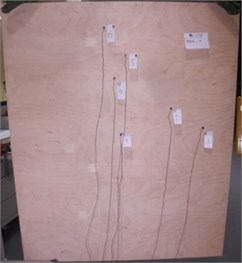a)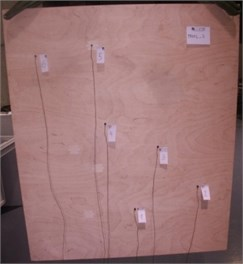b)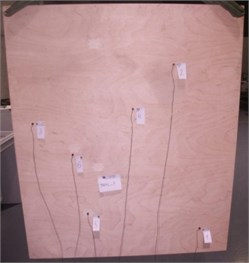c)

To ensure the highest decay signal variety (Fig. 1), for each plywood panel, the measurement could be repeated for three times, where the sensor can able to reposition during the measurement cycle.

Also, to ensure free-free modes of plywood panel, it can be hanged out with soft suspension.

## 3. Estimation

To estimate the 1/3 octave damping loss factor (DLF), the DRM methodology, also called T6 Reverberation time can be used. From the initial value to impact respond damping by 60 dB is measured T60 time of decay. For each frequency band, the decay time was evaluated and the damping is defined as:

1
$\eta =\frac{2.2}{f{T}_{60}}.$

The damping $\eta$ corresponds to the damping at 1/3 Octave Central Frequency $f$ and estimated time decay T60. Then 1/3 Octave filter is applied on each time signal per each frequency separately:

2
$x\left(t,{f}_{c},\mathrm{\Delta }f\right)=x\left(t\right)×h\left(t,{f}_{c},\mathrm{\Delta }f\right),$

where $x\left(t\right)$ is time domain input signal, $x$($t$, ${f}_{c}$, $\mathrm{\Delta }f$) is the filtered signal, $h$($t$, ${f}_{c}$, $\mathrm{\Delta }f$) is the transmissibility function of the used filter, $t$ is time, ${f}_{c}$ is the middle frequency of the band pass and $\mathrm{\Delta }f$ is the frequency step.

Fig. 2DRM estimation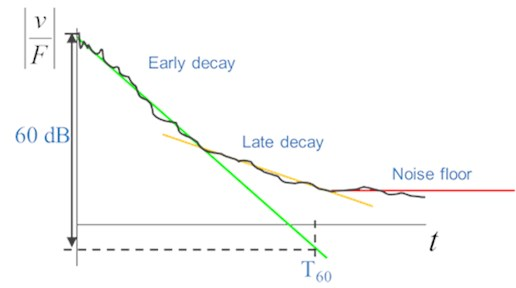The energy envelope can be created through filtered time signal, from the curve slope is determined as follow:

3
${e}^{2}\left(t,{f}_{c},\mathrm{\Delta }f\right)=\left(x\left(t,{f}_{c},\mathrm{\Delta }f\right)+x\left(t,{f}_{c},\mathrm{\Delta }f\right){\right)}^{2},$

where $e$ is the energy envelope:

4
$10\cdot \mathrm{l}\mathrm{o}{\mathrm{g}}_{10}\left({e}^{2}\left(t,{f}_{c},\mathrm{\Delta }f\right)\right).$

Evaluation is made at each sensor position independently. Positions of the sensors are chosen randomly in such way, that the maximum number of structural modes could be excited. Then hammer impact is applied at each sensor position. It populates 6 (6 accelerometers) response signals per one impact position, repeating this for six impact positions results in 36 DLF curves per single measurement. By averaging evaluated DLF’s curves, a final DLF curve is obtained:

5
$⟨\eta \left({f}_{c},\mathrm{\Delta }f\right)⟩=\frac{1}{MN}{\sum }_{i=1}^{M}{\sum }_{j=1}^{N}\left({\eta }_{ij}\left({f}_{c},\mathrm{\Delta }f\right)\right),$

where $M$ is the number of all the positions and $N$ is the number of impacts.

Six acceleration sensors can be available at measurement. For on configuration, set of measurement having six measurements. For future simulation, the final results of the average value of damping in 1/3 octave band and which has linearized damping representation can be used.

### 3.1. Anti-aliasing filtering of the force and acceleration signals

The sampling frequency was 500 kHz, and the aliasing filter was set to 10 kHz with a large reserve. The highest period 10 kHz is sampled as 50 samples. The maximum frequency is acceptable for the input impact by the hammer (relevant excitation is expected till 8 kHz). The filter is designed as low pass gate FIR.

### 3.2. Force and acceleration spectrum by using FFT

Impact hammer response depends on analyzed material behavior. The plywood composite impact force spectrum is applicable till 8 kHz.

Fig. 3Force magnitude spectrum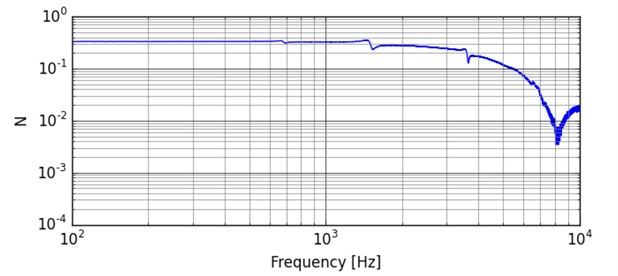### 3.3. FRF estimation from the Force and acceleration spectrum in complex form

FRF in complex form enables the correlation between non-equal impact levels. The maximum impact level is not possible to get the accurate repeatability.

Fig. 4FRF function as a spectrum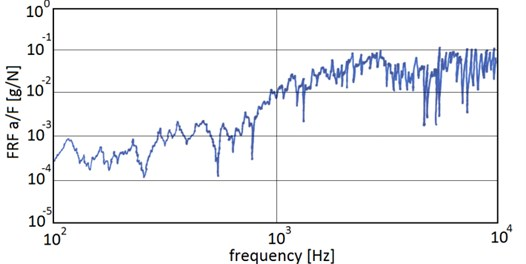### 3.4. Inverse FFT estimation – transformation of FRF from frequency to time domain

The Decay Rate Method is based on time domain signal. The inverse FFT for FRF enables the comparable magnitude to decrease in different impact levels.

### 3.5. Signal filtering across the 1/3 Octave band

The non-linear behavior and the frequency dependence of the loss factor require the estimation in the individual frequency bands. The IIR bandpass filter is used. Fig. 6. Describes the filter transmissibility function and the filtered signal for middle frequency 150 Hz.

Fig. 5FRF function in time domain form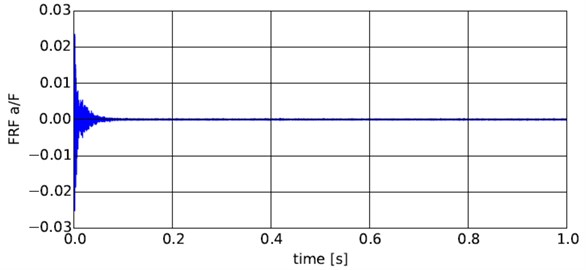Fig. 6Signal filtering across the 1/3 Octave band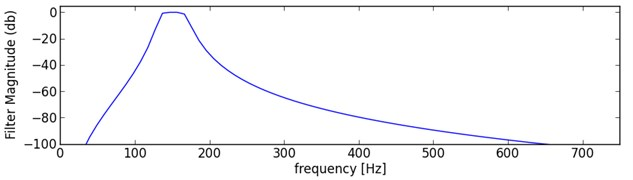a)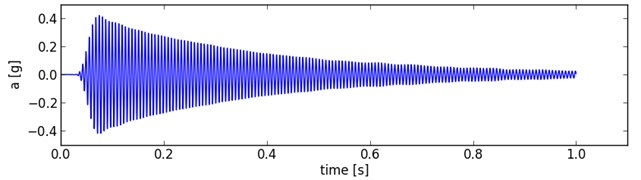b)

### 3.6. Envelope estimation of the early decay; Identification of the T60 in 60 dB decrease

The envelope of the time domain signal magnitude is used. The identification is performed on dB scale, where early decay slope is used to evaluate drop by 60 dB and estimate required time. There are significant differences between 1/3 octave bands (100, 1000, 4000 Hz middle band frequencies). The decrease is performed as the straight line. The straight line decrease deduction of the loss factor indicates the relevant estimation. The signal response for higher frequency bands shows the non-relevant estimation cause of the magnitudes close to the noise level.

The dispersion of the DLF estimation is in acceptable corridors till the whole spectrum described in Fig. 11. The faster damping also disperses the structural damping for higher frequencies. The lower frequency band dispersion affects the band pass filter cause of the relatively wide frequency step and the less kurtosis of the band stop.

The results show the significant dependency on frequency bands with DLF from 0.02 to 0.06.

Fig. 7Decay rate in 100 Hz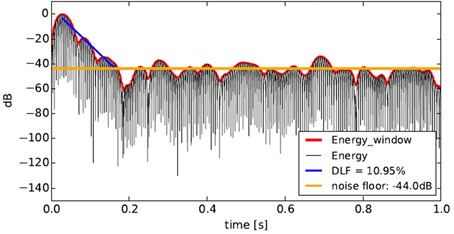Fig. 8Decay rate in 1000 Hz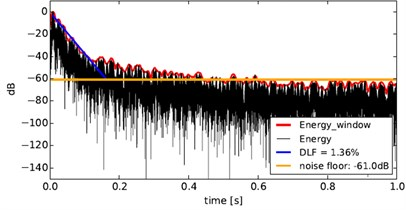Fig. 9Decay rate in 4000 Hz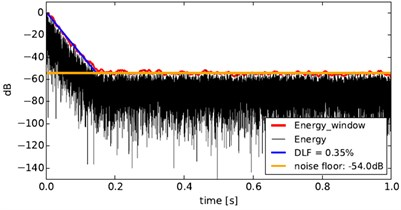Fig. 10DLF estimation across the 1/3 Octave band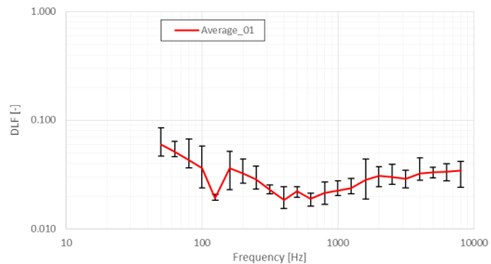Fig. 11DLF estimation across the 1/3 Octave band for different sensor distributions (Average_01, Average_02, Average_03)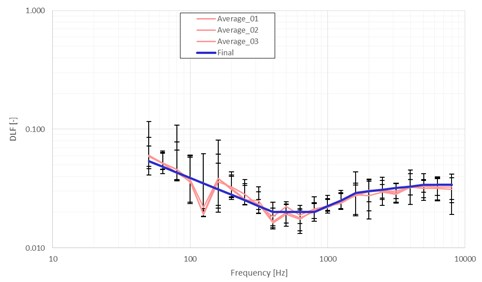## 4. Statistics

The statistics are suitable to analyze the relevant output of the sensors array. The loss factor dispersion is helpful information for the sensitivity analysis of the damping behavior. Dispersion of the loss factor influence panel vibrations and panel radiation, what consequently cause dispersion of the acoustic results. It is mandatory to use some stochastic method for the virtual simulation like the loss factor generation from the relevant distribution in finite element models.

The same input conditions are necessary for the statistics analysis. The loss factor estimation sensitivity depends on the sensor distribution. The loss factor estimation is performed for each of 6 hammer impacts for 5 accelerometer sensors. The statistic sample contains 30 values of the loss factor.

The statistical analysis is suitable to perform across the 1/3 Octave band for each sensor distribution.

The loss factor distribution for the lower frequency modes (lower than 200 Hz) evinces, the larger values. The loss factor histograms for 100 Hz and 1000 Hz are shown in Fig. 12.

Fig. 12DLF histogram for 100 Hz and 1000 Hz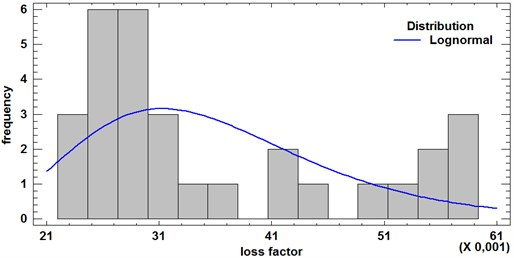a) 100 Hz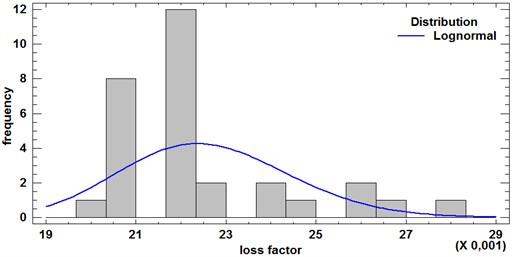b) 1000 Hz

Kolmogorov-Smirnov (also K-S) goodness of fit test has been used for determination of suitable theoretical distribution of measured data. It tests hypothesis ${H}_{0}$ which saying selected sample ${X}_{1}$, …, ${X}_{n}$ have a distribution $\mathrm{\Phi }\left(x\right)$. The statistics are computed using Eq. (6), where ${F}_{n}\left(x\right)$ is selective distribution.

6
${D}_{n}=\underset{-\infty

In case ${D}_{n}\ge {D}_{n}\left(\alpha \right)$, where ${D}_{n}\left(\alpha \right)$ is tabulated critical value, we can reject ${H}_{0}$ with the level of significance $\alpha$. In comparison with Pearson ${\chi }^{2}$ goodness of fit test, the advantage of K-S test lies in the possibility of usage also in the case of a small sample of data and in regard to empty classes of the histogram.

The K-S tests shown that the experimental values of loss factor come from a lognormal distribution which is given by Eq. (7):

7
$f\left(x\right)=\frac{1}{x\sigma \sqrt{2\pi }}\cdot \mathrm{exp}\left(-\frac{1}{2}{\left(\frac{\mathrm{l}\mathrm{n}x-\mu }{\sigma }\right)}^{2}\right),$

where $\mu$ (mean) and σ (standard deviation) are parameters of lognormal distribution $LN$ ($\mu$, $\sigma$). SW Statgraphics Centurion XVI has been used for introduced statistical analysis.

## 5. Application

The materials with DLF close to 0.1 in higher frequency spectrum caused the dispersion of DRM results. The dispersion of the estimated DLF is due to the higher damping of the panel above 2 kHz and the faster magnitude loss in time. The time-domain filtered signals of higher frequencies are estimated in the noise level, and it causes the result dispersion. The impact hammer pulse contains sufficient energy, but the panel response is more damped above 2 kHz. Case study example is described in Fig. 13. The sensitivity of used accelerometers is applicable for spectrum till 2 kHz. The limits of DRM is also with DLF > 0.05 for spectrums above 2 kHz.

The DRM results for layered plywood shows the dependency on the frequency with acceptable dispersion results. The advantage of this DRM methodology is the complex modeling of the layered materials, and there is no need to identify the separate layer. The plywood analysis is used for the acoustic analysis of the railway producer. The simulation results are confidential.

The application for the automotive industry might be the analysis of the acoustic comfort of the car crew.

The influence of side glass is the significant contribution to the overall acoustic pressure of the cavity inside the car body.

Fig. 13DLF estimation across the 1/3 Octave band with dispersion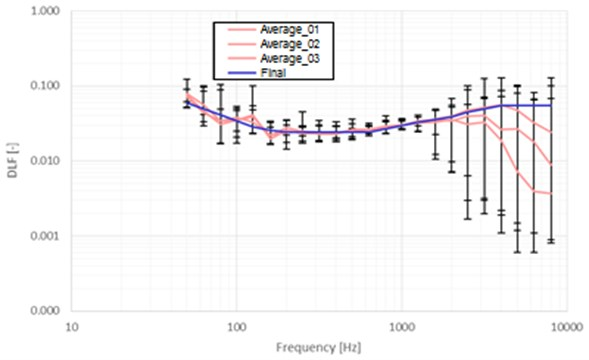In the wind noise simulation, where side glass panel is excited by very complex air flow mechanism, panel response becomes critical to passenger’s noise comfort. Panel damping fully controls how much energy is then radiated into the interior. The DRM method enables accurate prediction of the panel response and thus more precise interior cavity pressure prediction.

Fig. 14Side glass panel vibration at 630 Hz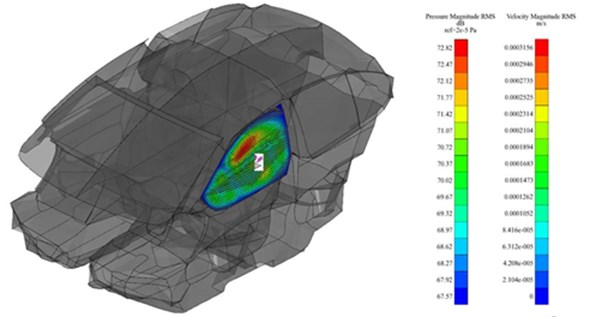Fig. 15Cavity direct field representation at 630 Hz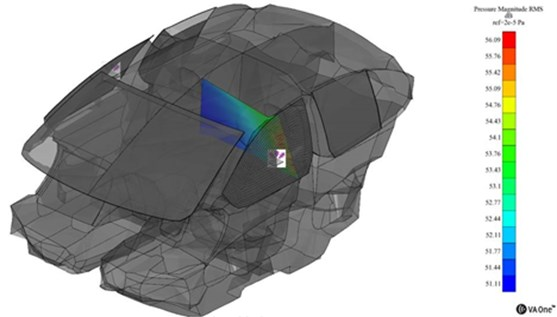## 6. Conclusions

The Decay Rate Method is applicable for common materials. The dispersion of the estimated result might be caused by the lower magnitude level in higher frequencies for the lower stiffness materials and the 1/3 octave IIR filter in lower frequencies by lower kurtosis. The method is not possible to use for soft material like polyurethane foams, polypropylene plastics, and others. There is the significant benefit what could be extracted from a couple of experimental measurement and damping analysis. The estimation requires the minimum statistical number of the measurements cause of the dispersion of impact force level and response.

The loss factor estimation of the plywood material is possible to get by using the Decay Rate Method. This method enables the easy identification of the plywood panel behavior with the impact hammer response close to the real conditions. The proper virtual analysis enables the stochastic designation of the DLF with the lognormal distribution. The relevant stochastic dispersion seems to be effective for the sensitivity analysis of the complex model or even on the separate part of the plywood panel behavior in term of the acoustic reduction analysis in a virtual environment. The dispersion of the DLF causes the sensitivity of used sensors like the distortion in the low band or the small signal to noise distance in high band frequencies and inhomogeneity of the plywood panel like the structural behavior dispersion.

#### Cited by

26 May 2017
Accepted
27 October 2017
Published
15 May 2018
SUBJECTS
Acoustics, noise control and engineering applications
Keywords
vibration
damping loss factor
plywood
Acknowledgements

This publication was written at the Technical University of Liberec as part of the project “Innovation of Products and Equipment in Engineering Practice” with the support of the Specific University Research Grant, as provided by the Ministry of Education, Youth and Sports of the Czech Republic in the year 2016.